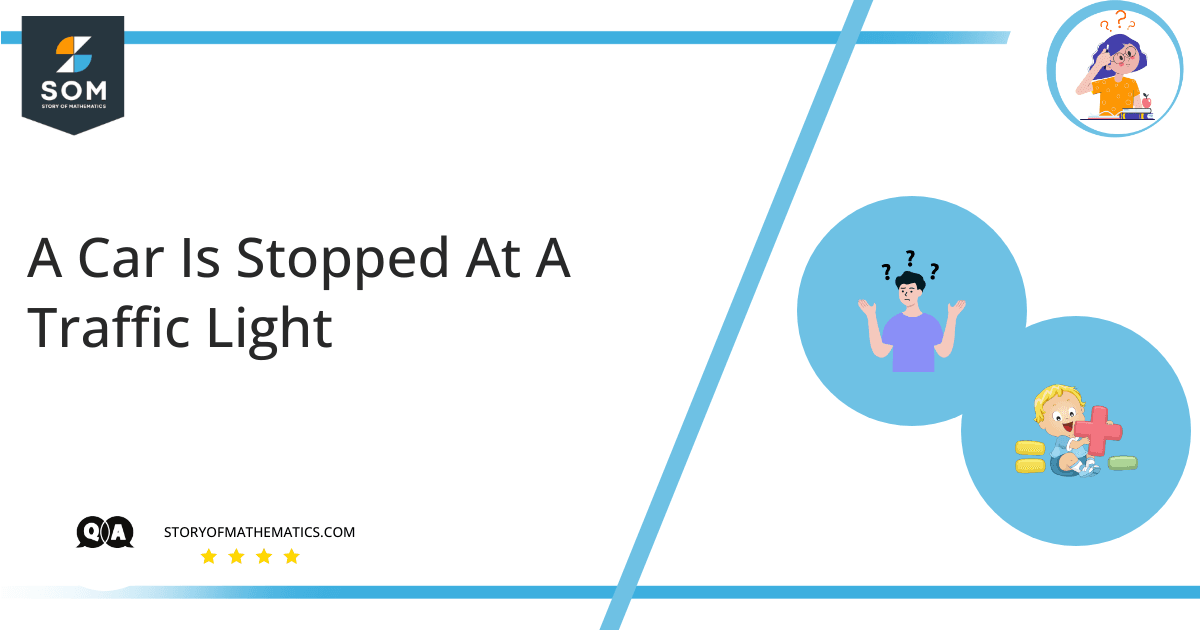# A car is stopped at a traffic light. It then travels along a straight road such that its distance from the light is given by x(t)=bt^2-ct^3, where b=2.40 m/s^2 and c=0.120 m/s^3. (a) Calculate the average velocity of the car for the time interval t = 0 to t = 10.0 s. (b) Calculate the instantaneous velocity of the car at t=0, t = 5.0 s, and t = 10.0 s. (c) How long after starting from rest is the car again at rest?This article aims to find a car’s average and instantaneous velocity at times. This article uses the concept of average velocity. Average velocity is the change in position $(\Delta x)$ divided by the time intervals $(\Delta t)$ in which the displacement occurs. Average velocity can be negative or positive depending on the sign of the shift. SI unit of average velocity is meters per second $(\dfrac{m}{s}\: or \:ms^{-1})$. The formula for average velocity is: $v_{avg} =\dfrac{\Delta x}{\Delta t}$

Distance from the light is represented by the equation $x(t) = bt ^ {2} – ct ^ {3}$. Where $b = 2.40 \dfrac{m}{s^{2}}$ and $c=0.120 \dfrac{m}{s^{3}}$. Part (a) Distance from the light is: $x (t) = bt ^ {2} – ct ^ {3}$ At $t=0$ $x(0)=b0 ^ {2} – c0 ^ {3}= 0m$ At $t=10s$ $x(t) = 2.40\times 10^{2} – 0.12 \times 10^{3}$ $x(t) = 2.40\times 100 – 0.12\times 1000$ $x(t) = 120m$ The average velocity of car for time interval $t = 0s$ and $t=10s$ is given by: $v_{avg}= \dfrac{dx}{dt}$ $= \dfrac{120-0}{10-0}$ $v_{avg}= 12\dfrac{m}{s}$ Average velocity of car for time interval $t=0s$ and $t=10s$ is $v_{avg}=12\dfrac{m}{s}$. Part (b) Instantaneous velocity of car at $t= 0s$; $t=5s$ and $t=10s$. $v_{x}=\dfrac{dx}{dt} = 2bt-3ct^{2}$ At $t=0$ $v_{x}= 2b(0) -3c (0)^{2}=0$ At $t=5s$ $v_{x} = 2\times 2.4\times 5- 3\times 0.12\times 25$ $= 15\dfrac{m}{s}$ At $t=10s$ $v_{x}= 2\times 2.4\times 10 – 3\times 0.12 \times 100$ $= 12\dfrac{m}{s}$ Instantaneous velocity at the time interval $t=0$;$t=5s$ and $t=10s$ are $v_{x}=0$,$v_{x}=15\dfrac{m}{s}$ and $v_{x}=12\dfrac{m}{s}$. Part (c) The car is at rest means that: $v_{x} = 0\dfrac{m}{s}$ Put the equation to zero, and we can find at what time the car will be at rest again. $2bt-3ct^{2}=0$ $t=\dfrac{2b}{3c}$ $t=\dfrac{2(2.40)}{3(0.120)}$ $t=13.33s$ The car will be at rest after $t=13.33s$.

## Numerical Result

– The average velocity of the car for the time interval $t=0s$ and $t=10s$ is $v_{avg}=12\dfrac{m}{s}$. – The instantaneous velocity of the car at $t=0$;$t=5s$ and $t=10s$ are $v_{x}=0$,$v_{x}=15\dfrac{m}{s}$ and $v_{x}=12\dfrac{m}{s}$. – The car will be at rest after $t=13.33s$.

## Example

The car is stopped at a traffic light. He then drives along a straight road such that his distance from the light is given $x(t)=bt^{2}+ct^{3}$, where $b=1.40\dfrac{m}{s^{2}}$ and $c=0.120\dfrac{m}{s^{3}}$. 1: Calculate the car’s average velocity at time interval $=5s$. 2: Find the instantaneous velocity of the car at time interval $t=5s$ and $t=10s$. Solution Distance from the light is shown by the equation $x(t) = bt ^ {2} + ct ^ {3}$. Where $b = 1.40 \dfrac{m}{s^{2}}$ and $c=0.120 \dfrac{m}{s^{3}}$. Part 1 Distance from the light is: $x(t) = bt ^ {2} + ct ^ {3}$ At $t=5s$ $x(t) = 1.40\times 5^{2} + 0.12 \times 5^{3}$ $x(t) = 1.40\times 25 + 0.12\times 125$ $x(t) = 50m$ Average velocity of car for time interval $t=5s$ is given by: $v_{avg}= \dfrac{dx}{dt}$ $= \dfrac{50-0}{5-0}$ $v_{avg}= 10\dfrac{m}{s}$ Part (b) Instantaneous velocity of the car at $t=5s$ and $t=10s$. $v_{x}=\dfrac{dx}{dt} = 2bt+3ct^{2}$ At $t=5s$ $v_{x} = 2\times 1.4\times 5 + 3\times 0.12\times 25$ $= 23\dfrac{m}{s}$ At $t=10s$ $v_{x}= 2\times 1.4\times 10 + 3\times 0.12 \times 100$ $= 64\dfrac{m}{s}$